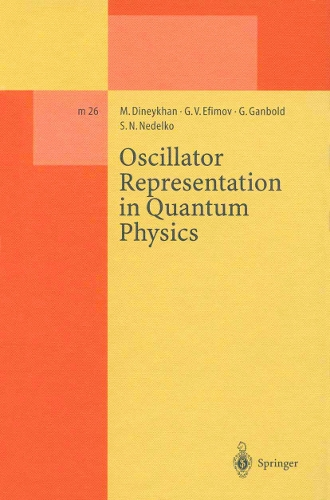Find your perfect holiday reading•# Oscillator Representation in Quantum Physics - Lecture Notes in Physics Monographs 26 (Paperback)

(author), (author), (author), (author)
£79.99
Paperback 282 Pages / Published: 18/04/2014
• We can order this

Usually dispatched within 3 weeks

• This item has been added to your basket
The investigation ofmost problems of quantum physics leads to the solution of the Schrodinger equation with an appropriate interaction Hamiltonian or potential. However, the exact solutions are known for rather a restricted set of potentials, so that the standard eternal problem that faces us is to find the best effective approximation to the exact solution of the Schrodinger equation under consideration. In the most general form, this problem can be formulated as follows. Let a total Hamiltonian H describing a relativistic (quantum field theory) or a nonrelativistic (quantum mechanics) system be given. Our problem is to solve the Schrodinger equation Hlft = Enlftn, n i. e. , to find the energy spectrum {En} and the proper wave functions {lft } n including the'ground state or vacuum lft = 10). The main idea of any ap- o proximation technique is to find a decomposition in such a way that Ha describes our physical system in the "closest to H" manner, and the Schrodinger equation HolJt. (O) = E(O)lJt. (O) n n n can be solved exactly. The interaction Hamiltonian HI is supposed to give small corrections to the zero approximation which can be calculated. In this book, we shall consider the problem of a strong coupling regime in quantum field theory, calculations ofpath or functional integrals over the Gaussian measure and spectral problems in quantum mechanics. Let us con- sider these problems briefly.

Publisher: Springer-Verlag Berlin and Heidelberg GmbH & Co. KG
ISBN: 9783662140635
Number of pages: 282
Weight: 456 g
Dimensions: 235 x 155 x 16 mm
Edition: Softcover reprint of the original 1st ed. 199

## Reviews

Sign In To Write A Review

Please sign in to write a review

Your review has been submitted successfully.